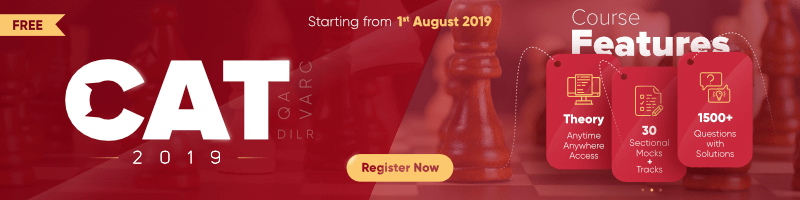# Probability

• Sample Space (S) : The set of all possible outcomes of an event
• Probability : The likeness of an event to happen
• Probability = Number of favorable outcomes / Total Number of outcomes
• 0 ≤ P ≤ 1

### Sample Problems

Question 1 : Three unbiased coins are tossed. What is the probability that atmost one head occurs ?
Solution : S = {HHH, HHT, HTH, THH, HTT, THT, TTH, TTT}
Favorable outcomes = {HTT, THT, TTH, TTT}
Total number of outcomes = 8
Number of favorable outcomes = 4
Required probability = 4 / 8 = 0.50

Question 2 : Find the probability of getting a red card when a card is drawn from a well shuffled pack of cards.
Solution : Total number of outcomes = 52
Number of favorable outcomes = Number of red cards = 26
=> Required probability = 26 / 52 = 0.50

Question 3 : A bag contains 6 white and 4 black balls. Two balls are drawn at random from the bag. Find the probability that both the balls are of the same color.
Solution : Outcome will be favorable if the two balls drawn are of the same color.
=> Number of favorable outcomes = 6 C 2 + 4 C 2 = 21
Total number of outcomes = 10 C 2 = 45
Therefore, required probability = 21 / 45 = 7 / 15

Question 4 : An unbiased die is tossed. Find the probability of getting an even number.
Solution : S = {1, 2, 3, 4, 5, 6}
Favorable outcomes = {2, 4, 6}
Required probability = 3 / 6 = 0.50

Question 5 : From a bag containing red and blue balls, 10 each, 2 balls are drawn at random. Find the probability that one of them is red and the other is blue.
Solution : Total number of outcomes = 20 C 2 = 190
Number of favorable outcomes = 10 C 1 x 10 C 1 = 100
Therefore, required probability = 100 / 190 = 10 / 19

### Problems on Probability | Set-2

Please write comments if you have any doubts related to the topic discussed above, or if you are facing difficulty in any question or if you would like to discuss a question other than those mentioned above.My Personal Notes arrow_drop_up

Article Tags :

4

Please write to us at contribute@geeksforgeeks.org to report any issue with the above content.